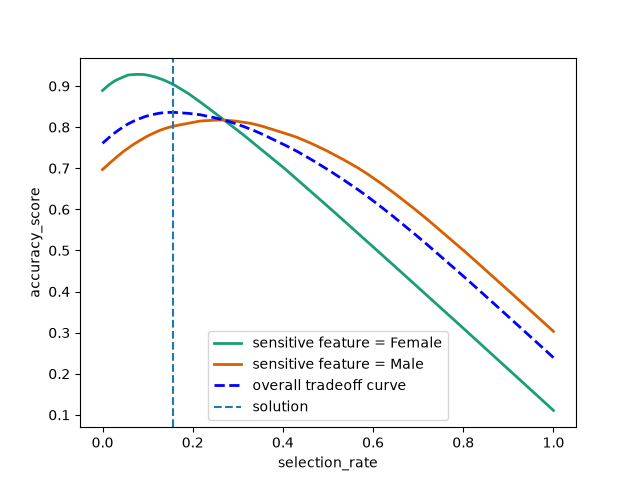# Passing pipelines to mitigation techniques#

This notebook shows how to pass `sklearn.pipeline.Pipeline` to mitigation techniques from Fairlearn. Note that the notebook is not to be used as an example for how to assess and mitigate fairness. It is merely a demonstration of the technical aspects of passing `sklearn.pipeline.Pipeline`. For more information around proper fairness assessment and mitigation please refer to the User Guide.

```import json

from sklearn.compose import ColumnTransformer
from sklearn.compose import make_column_selector as selector
from sklearn.impute import SimpleImputer
from sklearn.linear_model import LogisticRegression
from sklearn.model_selection import train_test_split
from sklearn.pipeline import Pipeline
from sklearn.preprocessing import OneHotEncoder, StandardScaler

from fairlearn.postprocessing import ThresholdOptimizer, plot_threshold_optimizer
```

Below we load the “Adult” census dataset and split its features, sensitive features, and labels into train and test sets.

```data = fetch_adult()
X_raw = data.data
y = (data.target == ">50K") * 1
A = X_raw["sex"]

(X_train, X_test, y_train, y_test, A_train, A_test) = train_test_split(
X_raw, y, A, test_size=0.3, random_state=12345, stratify=y
)

X_train = X_train.reset_index(drop=True)
X_test = X_test.reset_index(drop=True)
y_train = y_train.reset_index(drop=True)
y_test = y_test.reset_index(drop=True)
A_train = A_train.reset_index(drop=True)
A_test = A_test.reset_index(drop=True)
```

To illustrate Fairlearn’s compatibility with `Pipeline` we first need to build our pipeline. In the following we assemble a pipeline by combining preprocessing steps with an estimator. The preprocessing steps include imputing, scaling for numerical features and one-hot encoding for categorical features.

```numeric_transformer = Pipeline(
steps=[
("impute", SimpleImputer()),
("scaler", StandardScaler()),
]
)
categorical_transformer = Pipeline(
[
("impute", SimpleImputer(strategy="most_frequent")),
("ohe", OneHotEncoder(handle_unknown="ignore")),
]
)
preprocessor = ColumnTransformer(
transformers=[
("num", numeric_transformer, selector(dtype_exclude="category")),
("cat", categorical_transformer, selector(dtype_include="category")),
]
)

pipeline = Pipeline(
steps=[
("preprocessor", preprocessor),
(
"classifier",
LogisticRegression(solver="liblinear", fit_intercept=True),
),
]
)
```

Below we will pass the pipeline to some of our mitigation techniques, starting with `fairlearn.postprocessing.ThresholdOptimizer`:

```threshold_optimizer = ThresholdOptimizer(
estimator=pipeline,
constraints="demographic_parity",
predict_method="predict_proba",
prefit=False,
)
threshold_optimizer.fit(X_train, y_train, sensitive_features=A_train)
print(threshold_optimizer.predict(X_test, sensitive_features=A_test))
print(
json.dumps(
threshold_optimizer.interpolated_thresholder_.interpolation_dict,
default=str,
indent=4,
)
)
plot_threshold_optimizer(threshold_optimizer)
``````[0 0 1 ... 0 0 1]
{
"Female": {
"p0": 0.8004605263157903,
"operation0": "[>0.20325171890519633]",
"p1": 0.19953947368420966,
"operation1": "[>0.1873222488873632]"
},
"Male": {
"p0": 0.08521658986175128,
"operation0": "[>0.6820821878663272]",
"p1": 0.9147834101382487,
"operation1": "[>0.665722231006347]"
}
}
```

Similarly, `fairlearn.reductions.ExponentiatedGradient` works with pipelines. Since it requires the `sample_weight` parameter of the underlying estimator internally we need to provide it with the correct way of passing `sample_weight` to just the `"classifier"` step using the step name followed by two underscores and `sample_weight`.

```exponentiated_gradient = ExponentiatedGradient(
estimator=pipeline,
constraints=DemographicParity(),
sample_weight_name="classifier__sample_weight",
)
```[0 0 1 ... 0 0 1]